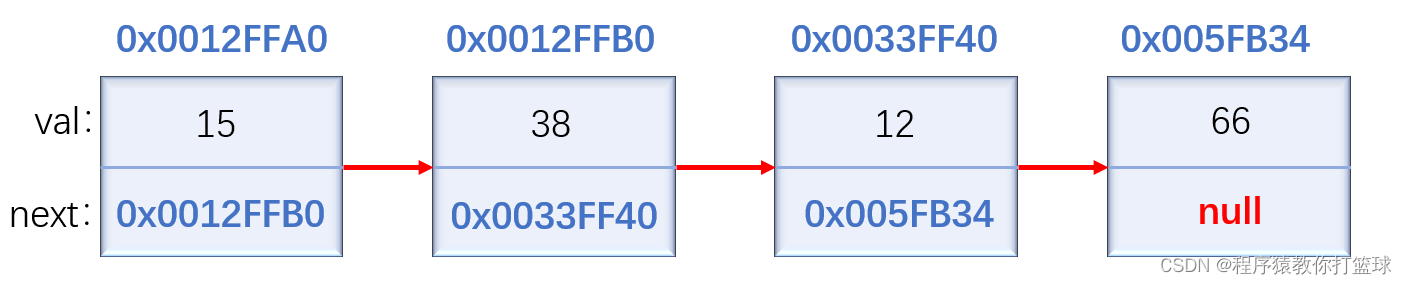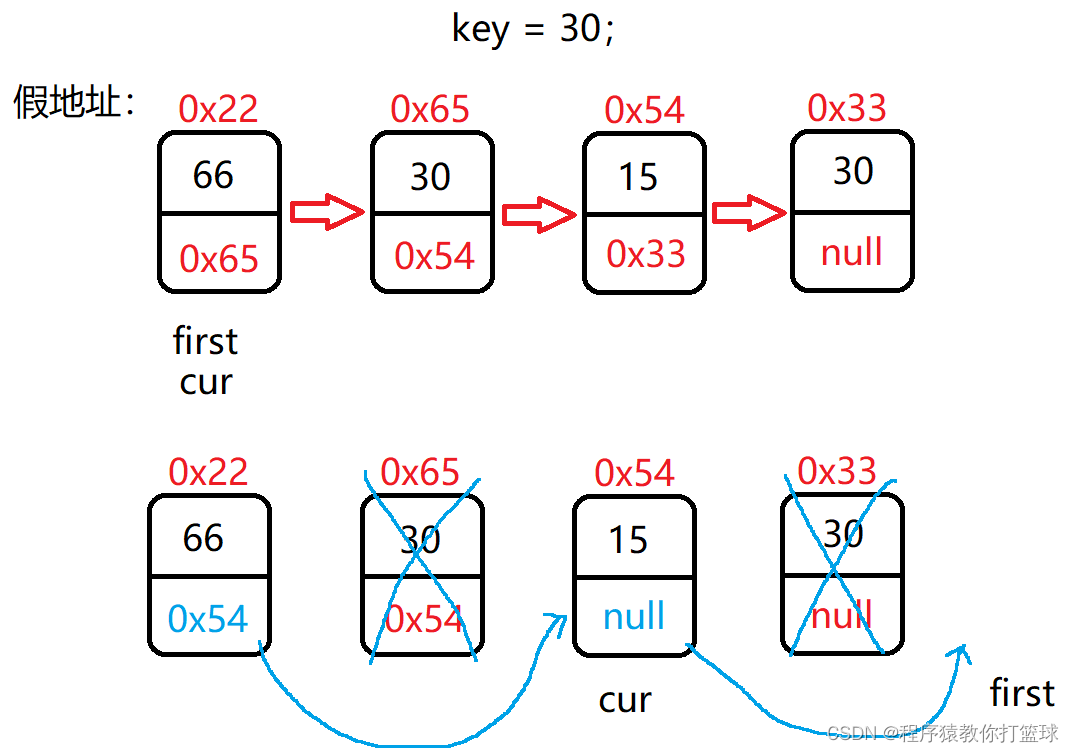> 文档中心 > 【Java 数据结构】单链表与OJ题

# 暮色降临🌃，冲一杯咖啡！

1、什么是链表

2、实现一个单向非循环链表

2.1 实现前的约定

2.5 contains 方法

2.6 remove 方法

2.7 removeAllKey 方法

2.8 size 和 clear 方法

3、单链表OJ题深度解剖

3.1 移除链表元素(来源:LeetCode 难度:简单)

3.2 反转链表(来源:LeetCode 难度:简单)

3.4 链表中倒数第k个节点(来源:牛客网 难度:简单)

3.6 链表分割(来源:牛客网 难度:较难)

3.7 链表的回文结构(来源:LeetCode 难度:较难)

3.8 相交链表(来源:LeetCode 难度:简单)

# 1、什么是链表？1. 单向、双向         2. 带头、不带头         3. 循环、非循环

# 2、实现一个单向非循环链表

## 2.1 实现前的约定

``public class MySingleList {    private ListNode head; //引用头节点    // 链表每个元素是一个节点    private class ListNode { private int val; //存放数据元素 private ListNode next; //存放下一个节点地址 //构造方法 public ListNode(int val) {     this.val = val; }    }}``

注意：链表最少有两个域，分别是数据域和指针域，当然你也可以有多个数据域和指针域。

``public void addFirst(int data); //头插法public void addLast(int data); //尾插法public boolean addIndex(int index,int data); //任意位置插入,第一个数据节点为0号下标public boolean contains(int key); //查找关键字key是否在单链表当中public void remove(int key); //删除第一次出现关键字为key的节点public void removeAllKey(int key); //删除所有值为key的节点public int size(); //得到单链表的长度public void clear(); //清空链表``

``public void addFirst(int data) {    ListNode newNode = new ListNode(data); //把传过来的值放到新的节点中    newNode.next = this.head; //新节点的next指向头节点    this.head = newNode; //使新节点成为头节点}``

``public void addLast(int data) {    ListNode newNode = new ListNode(data);    // 如果链表为空的情况    if (this.head == null) { this.head = newNode; return;    }    // 先找到最后一个节点    ListNode cur = this.head;    while (cur.next != null) { cur = cur.next;    }    cur.next = newNode;}``

``//任意位置插入,第一个数据节点为0号下标private ListNode findIndexPrevNode(int index) {    ListNode cur = this.head;    while (index - 1 != 0) { cur = cur.next; index--;    }    return cur;}public boolean addIndex(int index,int data) {    // 判断index下标的有效性    if (index  size()) { return false;    }    // 如果在0下标插入则是头插    if (index == 0) { addFirst(data); //头插 return true;    }    // 如果在末尾插入则是尾插    if (index == size()) { addLast(data); //尾插 return true;    }    ListNode newNode = new ListNode(data); //新节点    // 在中间插入    ListNode prevNode = findIndexPrevNode(index); //找到index下标的前一个节点    newNode.next = prevNode.next; //新节点的next被改为index的位置的节点    prevNode.next = newNode; //index位置前一个节点next被改成新节点    return true;}``

## 2.5 contains 方法

``//查找关键字key是否在单链表当中public boolean contains(int key) {    // 当链表为空的情况    if (this.head == null) { return false;    }    ListNode cur = this.head;    // 遍历列表    while (cur != null) { if (cur.val == key) {     return true; //找到了返回true } cur = cur.next;    }    return false; //找不到返回false}``

## 2.6 remove 方法

``//删除第一次出现关键字为key的节点public void remove(int key) {    if (this.head == null) { return;    }    ListNode cur = this.head;    // 如果删除的是key为头节点    if (this.head.val == key) { this.head = head.next; return;    }    // 这里不能是cur!=null, 不然会越界！！！    while (cur.next != null) { // 找到 key 的前一个节点 if (cur.next.val == key) {     //当前的cur为key的前一个节点     cur.next = cur.next.next; //cur链接到key的后一个节点     return; } cur = cur.next;    }}``

## 2.7 removeAllKey 方法

``//删除所有值为key的节点public void removeAllKey(int key) {    if (this.head == null) { return;    }    // 采用前后指针的方法    ListNode cur = this.head;    ListNode prev = this.head;    while (cur != null) { if (cur.val == key) {     prev.next = cur.next; //跳过key节点指向下一个节点 } else {     prev = cur; } cur = cur.next;    }    // 判断第一个节点是不是key    if (this.head.val == key) { this.head = this.head.next; //head指向下一个节点    }}``

# 3、单链表OJ题深度解剖

## 3.1 移除链表元素(来源:LeetCode 难度:简单)``public ListNode removeElements(ListNode head, int val) {    if (head == null) { return null;    }    ListNode cur = head;    ListNode first = head;    while (first != null) { if (first.val == val) {     cur.next = first.next; } else {     cur = first; } first = first.next;    }    // 判断头节点的值是否也是val    if (head.val == val) { head = head.next;    }    return head;}``

## 3.2 反转链表(来源:LeetCode 难度:简单)

``public ListNode reverseList(ListNode head) {    // 空链表的情况    if (head == null) { return null;    }    ListNode cur = head;    ListNode curNext = cur.next;    head.next = null;    while (curNext != null) { cur = curNext; curNext = curNext.next; cur.next = head; head = cur;    }    return head;}``

## 3.4 链表中倒数第k个节点(来源:牛客网 难度:简单)

``public ListNode FindKthToTail(ListNode head,int k) {    // 判断k的合法性    if (k <= 0 || head == null) { return null;    }    ListNode first = head;    ListNode slow = head;    // 先让first走k步    while (k != 0) { // k的长度大于链表的长度 if (first == null) {     return null; } first = first.next; k--;    }    // 一起走,当first为null,slow就是倒数第k个节点    while (first != null) { first = first.next; slow = slow.next;    }    return slow;}``

## 3.6 链表分割(来源:牛客网 难度:较难)

`` public ListNode partition(ListNode pHead, int x) { if (pHead == null) {     return null; } ListNode headA = null; ListNode headB = null; ListNode curA = null; ListNode curB = null; ListNode cur = pHead; while (cur != null) {     if (cur.val < x) {  // 第一次放入A盘子  if (headA == null) {      headA = cur;      curA = cur;  } else {      curA.next = cur;      curA = cur;  }     } else {  // 第一次放入B盘子  if (headB == null) {      headB = cur;      curB = cur;  } else {      curB.next = cur;      curB = cur;  }     }     cur = cur.next; } // 如果A盘子为空 if (headA == null) {     return headB; } curA.next = headB; // 避免B盘子尾节点形成环 if (headB != null) {     curB.next = null; } return headA;    }``

## 3.7 链表的回文结构(来源:LeetCode 难度:较难)

``public boolean chkPalindrome(ListNode A) {    if (A == null) { return false;    }    // 只有一个节点的情况    if (A.next == null) { return true;    }    // 首先找到中间节点    ListNode first = A;    ListNode slow = A;    while (first != null && first.next != null) { first = first.next.next; slow = slow.next;    }    // 走到这,slow是链表的中间节点,采用头插法反转slow后续的节点    first = slow.next;    ListNode cur = slow;    while (first != null) { cur = first; first = first.next; cur.next = slow; //链接前一个节点 slow = cur; //更新头节点的位置    }    // 走到这,反转完毕,cur指向最后一个节点,让slow等于A,往中间找    slow = A;    while (slow != cur) { if (slow.val != cur.val) {     return false; } // 偶数的情况下需要特殊判断 if (slow.next == cur) {     return true; } slow = slow.next; cur = cur.next;    }    return true;}``

## 3.8 相交链表(来源:LeetCode 难度:简单)

``public ListNode getIntersectionNode(ListNode headA, ListNode headB) {    if (headA == null || headB == null) { return null;    }    ListNode longList = headA; //longList始终记录长的链表    ListNode shortList = headB;    // 分别求出两个链表的长度    int lenA = 0;    int lenB = 0;    ListNode cur = headA;    while (cur != null) { lenA++; cur = cur.next;    }    cur = headB;    while (cur != null) { lenB++; cur = cur.next;    }    int len = lenA - lenB;    // 如果B链表长于A链表    if (len < 0) { // 修正相差长度 len = lenB - lenA; longList = headB; //longList始终记录长的链表 shortList = headA;    }    // 让长链表先走差值len步,然后同步走,相等了即为相交节点    while (len != 0) { longList = longList.next; len--;    }    while (longList != shortList) { longList = longList.next; shortList = shortList.next;    }    // 如果两个链表走到了null,则没有中间节点返回null,如果有,返回任意一个即可    return longList;}``